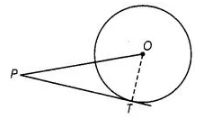# The length of tangent from an

Question:

The length of tangent from an external point P on a circle with centre 0 is always less than OP.

Solution:

True$P T$ is a tangent drawn from external point $P$. Join $O T$.

So, OPT is a right angled triangle formed.

In right angled triangle, hypotenuse is always greater than anv of the two sides of the triangle.

$\therefore$$Q P>P I$

or \$P T